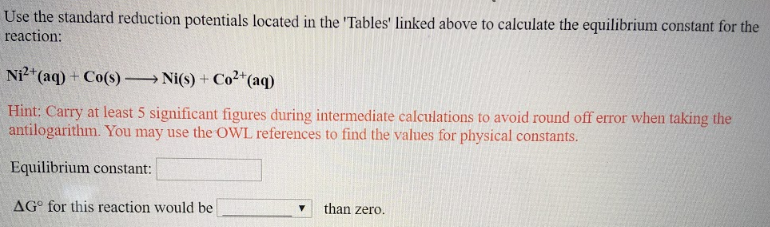# Use the table 'Standard Reduction Potentials' located in the 'Tables' linked above to calculate the equilibrium constant for the reaction: Ni^2+(aq) + Co(s) → Ni(s) + Co^2+(aq) Hint: Carry at least 5 significant figures during intermediate calculations to avoid round off error when taking the antilogarithm. You may use OWL references to find the values for physical constants. Equilibrium constant:______ ΔG° for this reaction would be ______ than zero.